# thesIt

•#### Arif 03:07:29 pm on August 24, 2010 | 0 | # | Tags: error, Geman 1992, MSE, neural network, poor data, Silvert 1998, variance

Bias-variance dilemma (Geman et al., 1992). It can be demonstrated that the mean square value of the estimation error between the function to be modelled and the neural network consists of the sum of the (squared) bias and variance. With a neural network using a training set of fixed size, a small bias can only be achieved with a large variance (Haykin, 1994). This dilemma can be circumvented if the training set is made very large, but if the total amount of data is limited, this may not be possible.

• #### Arif 12:41:37 pm on August 22, 2010 | 0 | # | Tags: cross-validation, error, estimation, k-fold, Michaelsen 1987, reference, Stone 1974

Stone 1974 is referenced in:
Michaelsen J. 1987. Cross-validation in statistical climate forecast models. J Climate Applied Meteorology, 26:1589-1600

1520-0450(1987)026-1589-cviscf-2.0.co;2.pdf

Set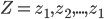$Z = z_{1}, z_{2},... , z_{1}$ consists of predictions and targets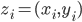$z_{i}=(x_{i}, y_{i})$

A set of prediction rule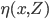$\eta (x,Z)$ will be used to predict y0 from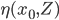$\eta (x_{0},Z)$

Let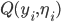$Q(y_{i},\eta_{i})$ be the accuracy.
by least squares this will usually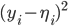$(y_{i}-\eta_{i})^{2}$
in other words expected Err is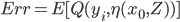$Err= E[Q(y_{i},\eta(x_{0},Z))]$

## MSE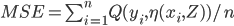$MSE= \sum_{i=1}^{n}Q(y_{i},\eta(x_{i},Z))/n$

In cross validation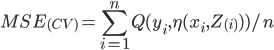$MSE_{(CV)}= \sum_{i=1}^{n}Q(y_{i},\eta(x_{i},Z_{(i)}))/n$

• #### Arif 12:19:08 pm on August 22, 2010 | 0 | # | Tags: basic, error, residual error, statistical error, wikipedia

If we assume a normally distributed population with mean μ and standard deviation σ, and take sample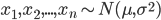$x_{1}, x_{2},..., x_{n}\sim N(\mu,\sigma^{2})$

statistical error is then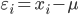$\varepsilon_{i}=x_{i}-\mu$

## Residual

while residual is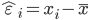$\hat{\varepsilon}_{i}=x_{i}-\bar{x}$

hat over the letter ε indicates an observable estimate of an unobservable quantity called ε.